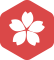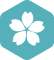### q=(NODE*)malloc(sizeof(NODE))

C/C++ > C语言 [问题点数：20分，结帖人puzzle1986]2008年6月 C/C++大版内专家分月排行榜第一
2008年5月 C/C++大版内专家分月排行榜第一
2008年4月 C/C++大版内专家分月排行榜第一2009年7月 C/C++大版内专家分月排行榜第三
2009年2月 C/C++大版内专家分月排行榜第三2008年9月 C/C++大版内专家分月排行榜第一
2008年8月 C/C++大版内专家分月排行榜第一puzzle1986

C++中的malloc与new分配内存空间（Qt中使用malloc时出错）

c语言中定义结构体指针并指向一片内存空间和直接定义一个结构体变量的...(Node *)malloc(sizeof(Node))...

Node *p是定义一个Node结构体指针，指针名为p,指向一个Node结构体，并为这个结构体分配了内存空间 该结构体无命名 Node p是定义一个Node结构体，结构体名为p。 追问：那两个的用法有什么区别？？？在...

p = (Node*)malloc(sizeof(Node));和 L = (CreateList)malloc(sizeof(Node));这个有什么区别？

t = (Node*)malloc(sizeof(Node)); t->data = m; s->next->next = t; s = t; s->next = NULL; } else { t = (Node*)malloc(sizeof(Node)); t->data = m; t->next = s->next; s->...

{ _q=(NODE *)malloc(sizeof(NODE));_ q->data=a[i]; q->next = NULL; if (h == NULL) h = p = q; else { p->next = q; p = q; } } return h; } void outlist(NODE *h) { NODE *p; p=h; if (p==NULL) ...

malloc sizeof 问题1： 1. L.elem = (ElemType *)malloc(LIST_INIT_SIZE*sizeof(ElemType)); 2. newbase = (ElemType *)realloc(L.elem,(L.listsize+LISTINCREMENT)*sizeof(ElemType)); 其中L是已经定义的...

sizeof函数计算数据（包bai括数组、变量、du类型、结构体等）所占内存空间，用zhi字节数表示。 malloc函数用于在内存开辟了一段地址，而这段地址的首地址存在返回的那个指针变量里，由于不知道到底这段地址有多长，...

C语言求教merge(struct node *p,struct node *q)哪里出错了？

h=p=(struct node*)malloc(sizeof(struct node)); for(i=0;i;i++) { q=(struct node*)malloc(sizeof(struct node)); q->data=a[i]; p->next=q; p=q; } p->next=0; return h; } void output(struct ...

Node * a 与Node* &a

struct Node{  int a;  int length; } int main(){  Node* a;  initNode(a);  return 0; } 错误方法：  void initNode(Node *a){ ... a = (Node*)malloc(sizeof(Node));  a->l

C语言 incompatible types - from 'struct nOde *' to 'struct node *

C语言中Node * a，和Node* &a的区别

*和*&之间的用法

c语言-链表实现

malloc初始化链表出现的错误

#include #include /* 链表节点的定义： */ struct node { int data;//节点内容 node *next;//下一个节点 }; //创建单链表(带有头结点） struct node *create() { int i=0;//链表中数据

//本人实在太懒了，没写一行注释，哈哈，没时间写，只是一个课堂练习///////////////////////////////////////////////////////////////////////////////////////makefile文件OBJS=mainApp.o mini_malloc.omalloc : ...

c/c++基础知识记录

11.求循环节

11.求循环节 成绩 10 开启时间 2014年11月12日 Wednesday 18:25 折扣 0.8 折扣时间 2014年11月30日 Sunday 23:55 允许迟交 ...对于任意的真分数 N/M

[C/C++基础知识] 那些被遗忘的链表知识

Node*p1 = (Node*)malloc(sizeof(Node)); if (p1 == NULL) { cout 申请内存失败" ; exit(0); } memset(p1, 0, sizeof(Node)); cout 输入城市名称： "; scanf_s("%s", p1->cityName, 20); cout 输入城市X...

1、单链表的建立、测长、打印 单链表建立可以正序建立或者逆序建立。 typedef struct node { int data; struct node *next;...node * Create(node *head, int n) { node *p; for(int i=0; i; i++) { p = (no